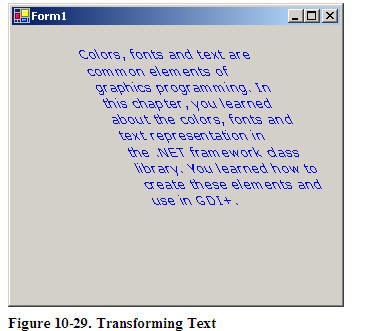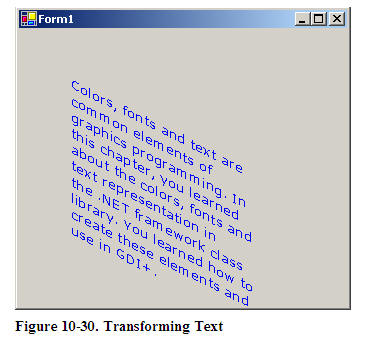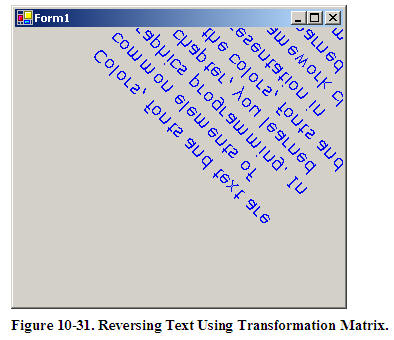# Text Transformation using GDI+ and C#

In Chapter 5, we discussed how to use the ScaleTransform, RotateTransform, and TranslateTransform methods to transform text. We can also use a transformation matrix to transform text.

We create a Matrix object with the transformation properties and apply it on the surface using the Transform property of the Graphics object. Listing 10-19 creates a Matrix object and sets it as the Transform property. We then call DrawString, which draws the text on the form. To test this code, add the code to a Form's paint event handler.

Graphics g = e.Graphics;

string str = "Colors, fonts and text are common" +" elements of graphics programming." + "In this chapter, you learned " +" about the colors, fonts and text" +" representation in the "+".NET framework class library. "+ "You learned how to create "+"these elements and use in GDI+.";
// Create a Matrix
Matrix M = new
Matrix(1, 0, 0.5f, 1, 0, 0);
g.RotateTransform(45.0f,
System.Drawing.Drawing2D.MatrixOrder.Prepend);
g.TranslateTransform(-20, -70);
g.Transform = M;
g.DrawString(str,
new
Font("Verdana", 10),
new
SolidBrush(Color.Blue),
new
Rectangle(50,20,200,300) );

Listing 10-19. Text Transformation Sample

Listing 10-19 Generates Figure 10-29.You can apply shearing and other effects by changing the values of Matrix. For example, if you change Matrix as follows:

Matrix M = new Matrix(1, 0.5f, 0, 1, 0, 0);

The new code will generate Figure 10-30.Figure 10-30. Transforming Text

We can reverse the text by just changing the value of the Matrix object as follows:

Matrix M = new Matrix(1, 1, 1, -1, 0, 0);

Wit results shown in Figure 10-31.This articles is taken from Chapter 10 "Transformations" of Graphics Programming with GDI+.

Mindcracker
Founded in 2003, Mindcracker is the authority in custom software development and innovation. We put best practices into action. We deliver solutions based on consumer and industry analysis.